Home » Posts tagged "task cards"

FREE Football Task Cards For Subtracting Money (Coins)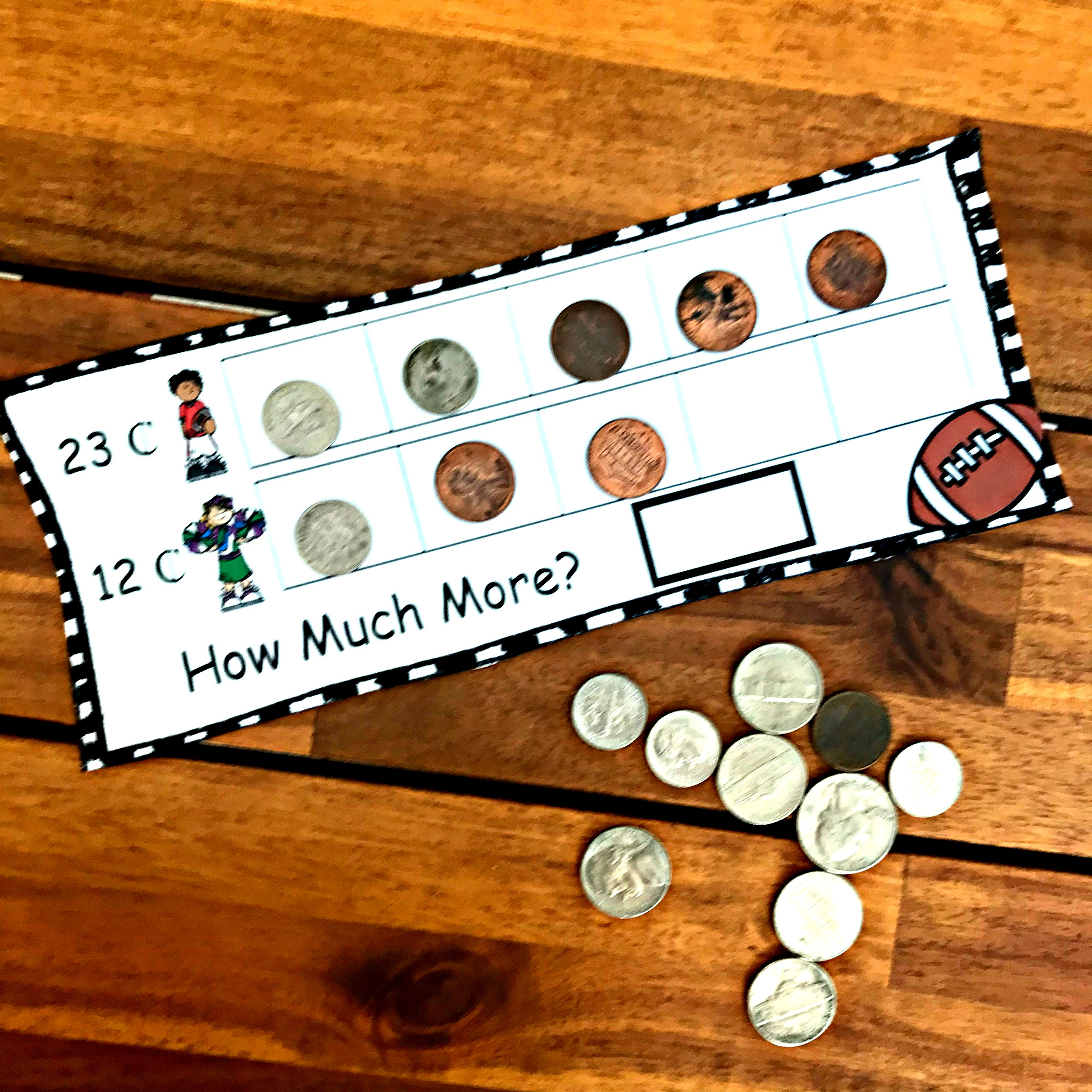Your little ones will love this subtracting money activity. It is a simple introduction to subtracting coins and answering the question, “How much more?” It is football season, and my little five-year-old is loving playing football with his older brothers. So when I noticed that...

How to Model Multiplying Decimals With Arrays (Free Printable)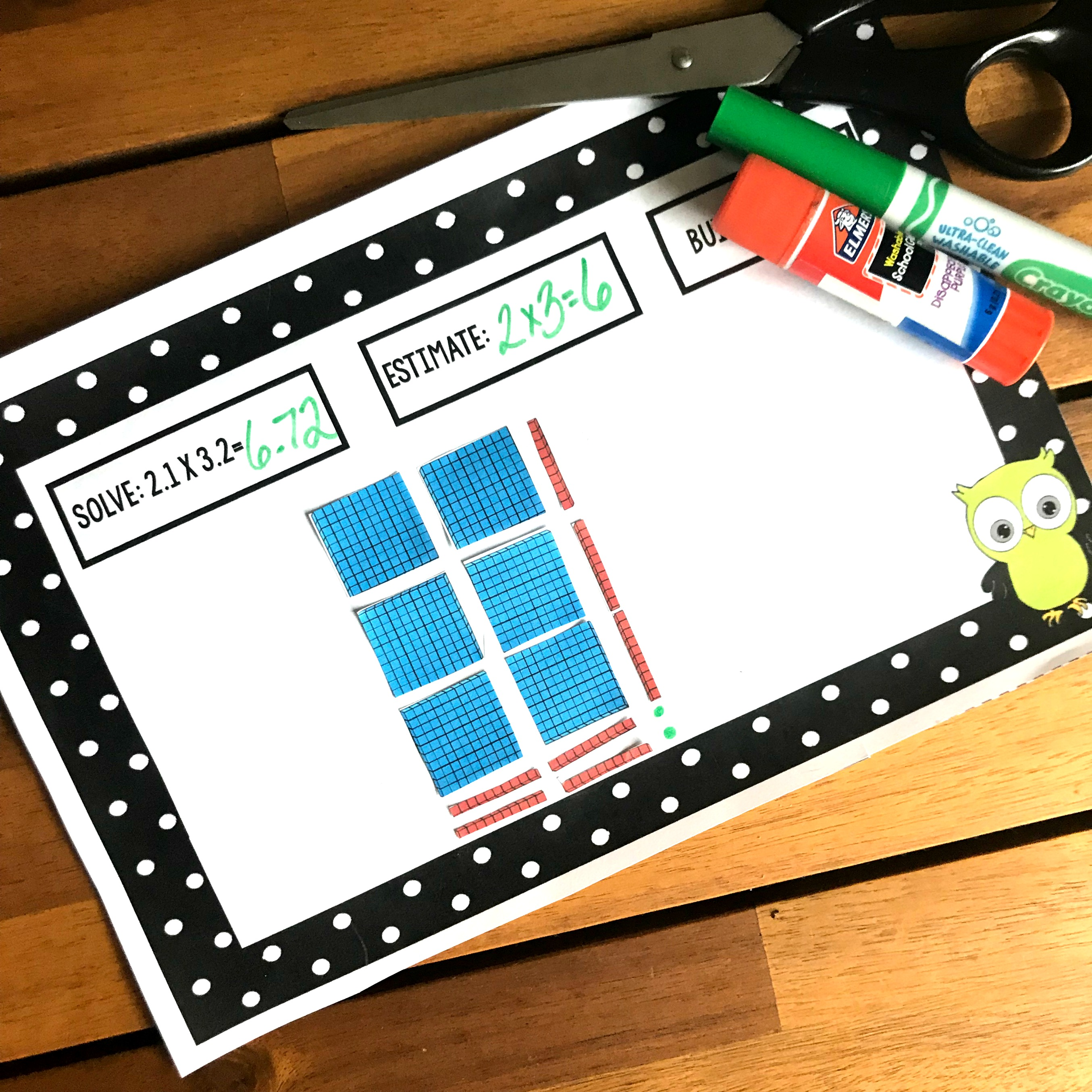This fun interactive worksheet has children learning how to model multiplying decimals with arrays. It’s a great way for children to visualize what is happening when they multiply decimals. I have very strong feelings on how math should be taught. And if you have read...

FREE Subtracting Decimals Activity With Base Ten Blocks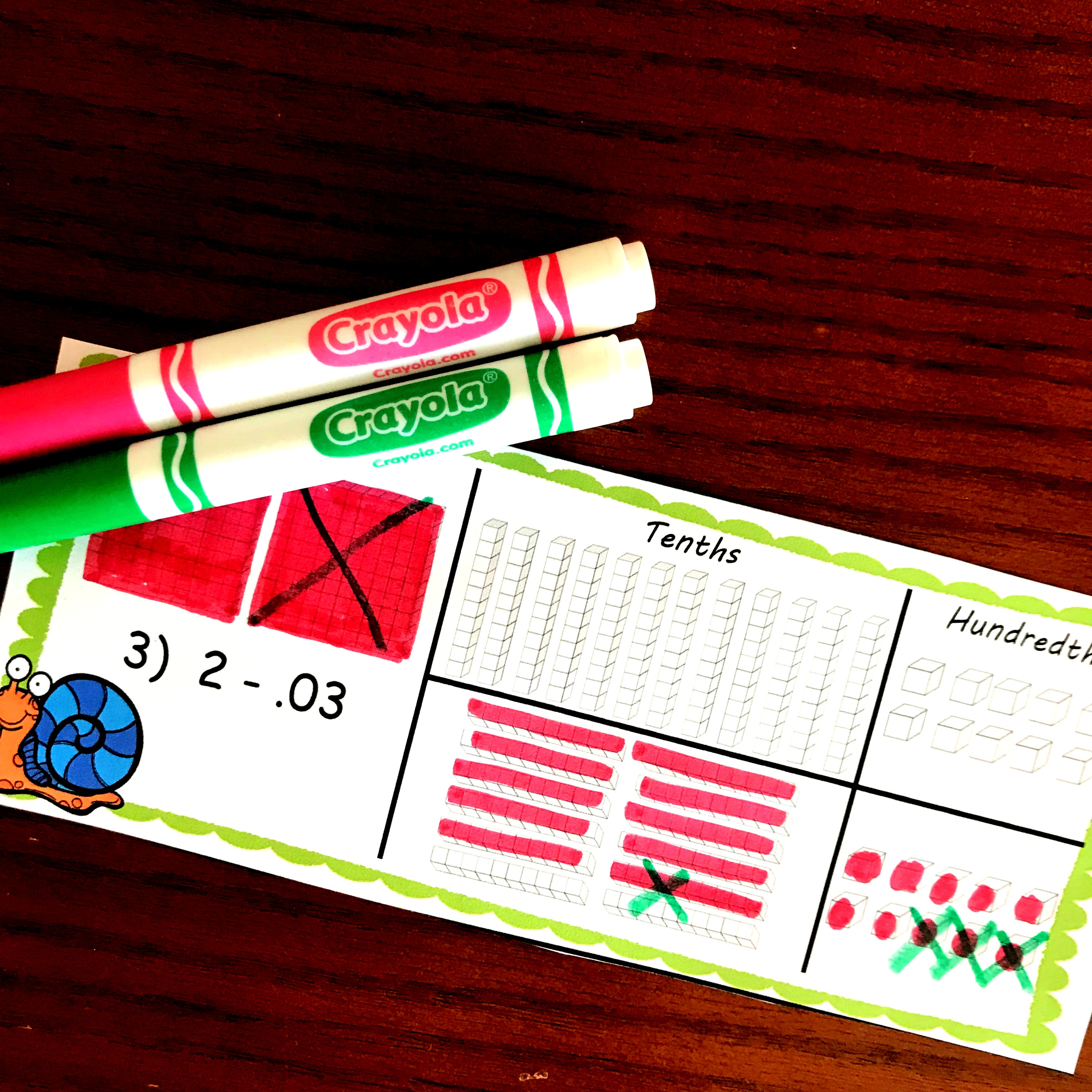Do you have a child struggling with subtracting decimals? This subtracting decimals activity works on regrouping with base ten blocks. Subtracting with regrouping is just plain hard. But when you add decimals…..it gets a little crazier. Subtracting 1 – .56 requires students to have an...

FREE Bright and Fun Task Cards To Multiply Decimals with ModelsPractice how to multiply decimals with models using these FREE task cards. Children will color in decimal grids to solve problems, as well as create equations based on a colored in a decimal grid. You can get the FREE printable by clicking on the large blue...

16 Task Cards To Practice Modeling Decimals on Number Lines and Decimal GridsThese task cards help children with modeling decimals. One set has children coloring a decimal grid to represent a decimal, while another has children figuring out the decimal represented on a decimal grid. The other two sets have children modeling decimals on number lines.  ...

Here’s FREE Missing Factor Worksheets or Task Cards For Extra Practice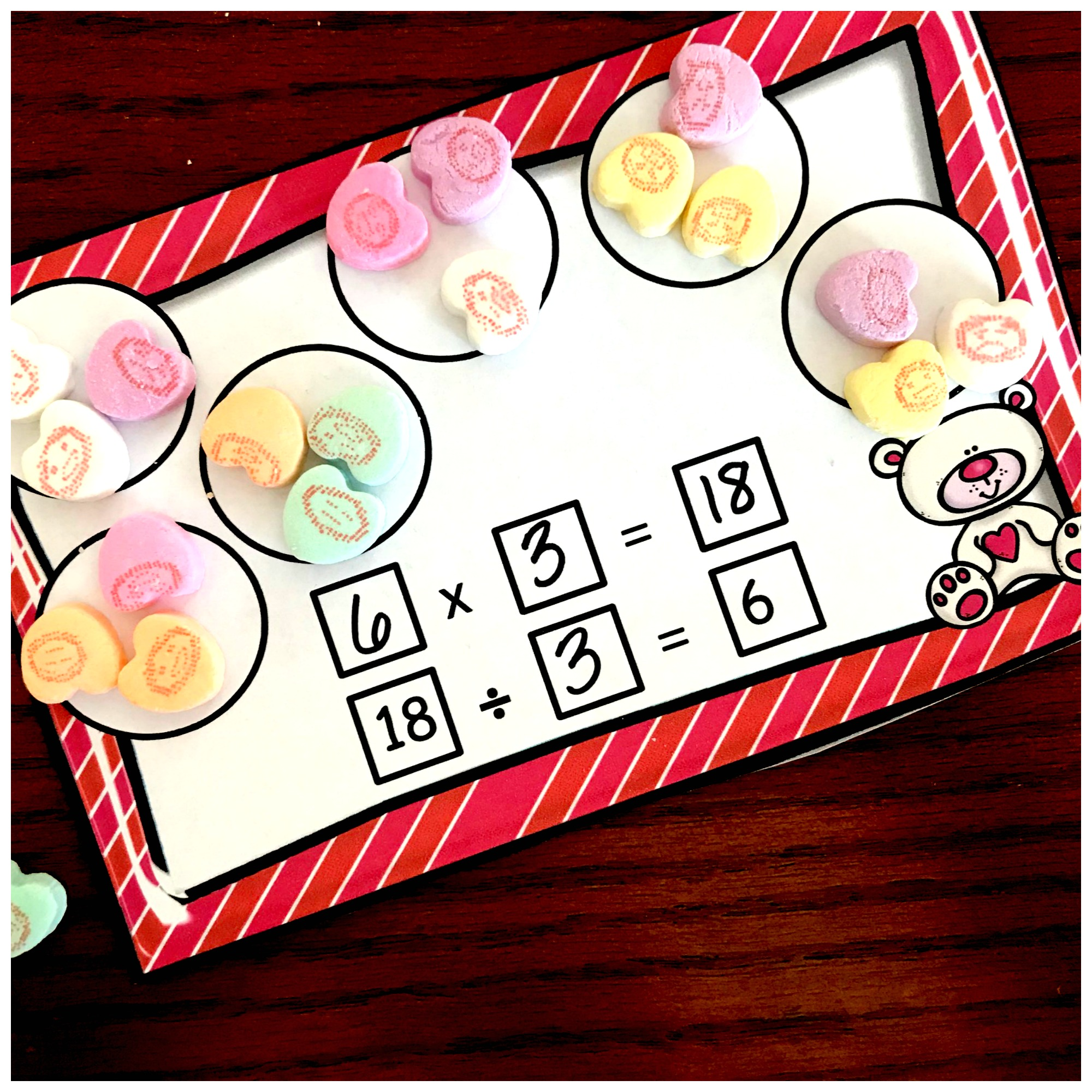These missing factor worksheets or task cards use conversation hearts to help children solve equations with missing factors, dividends, and divisors. Math was not easy for me in high school. I didn’t understand place value, and absolutely no fraction sense, and sat through most of...

Fun, Hands-On Task Cards For Teaching Missing Numbers in EquationsThese task cards are a great way to help children practice filling in missing numbers in equations. The ten frames and manipulatives help kiddos struggling in math to be successful. Grab this free printable by clicking on the bluish button at the bottom of the...

Grab your FREE Estimating Length Worksheets For Centimeters and Inches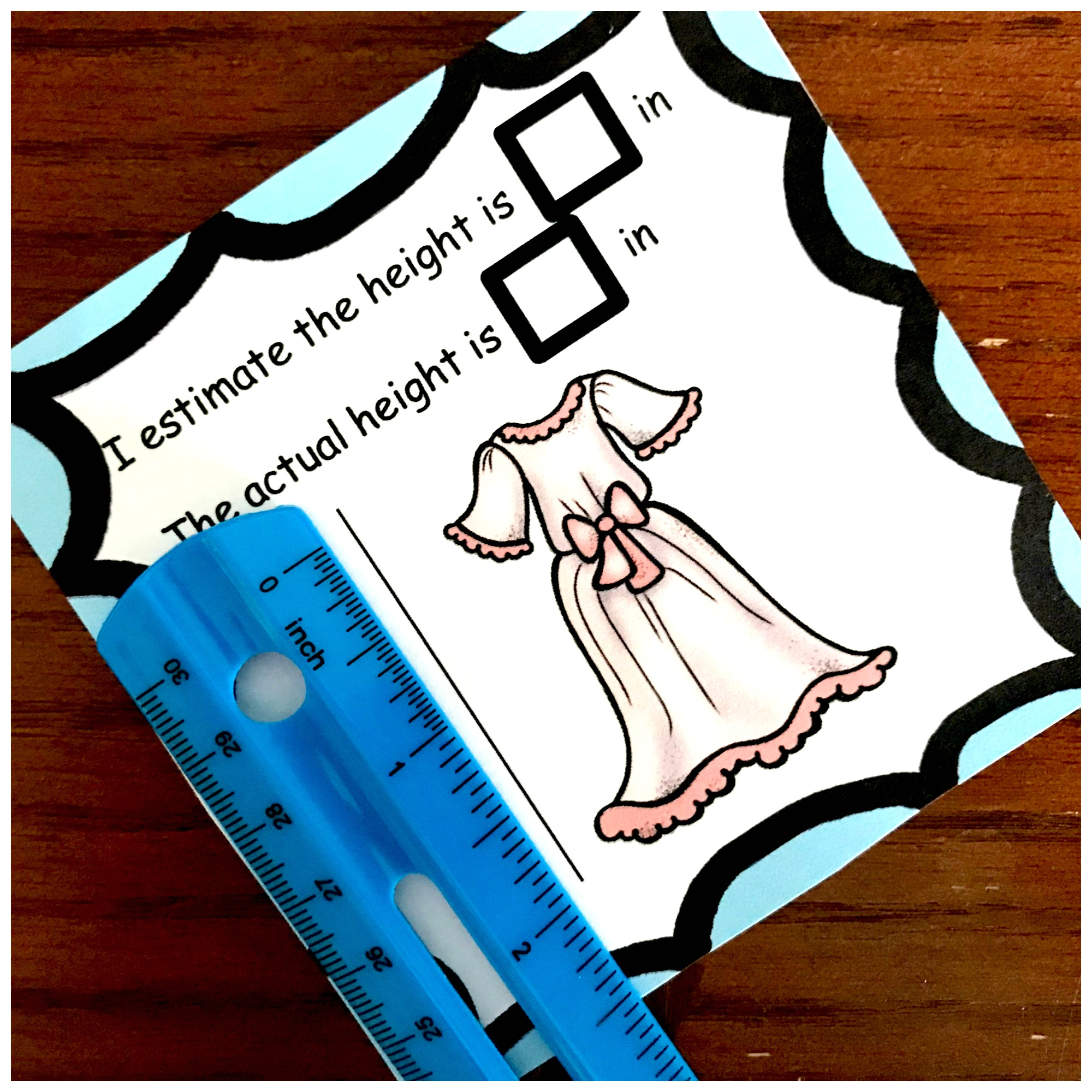These FREE estimating length worksheets or task cards are the perfect way for children to estimate small lengths in centimeters and inches. They then check their estimation using a ruler. *This post contains affiliate links There are so many aspects of measuring. You have measurement conversions,...